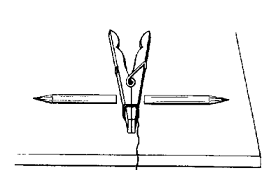## An experiment with force and motion, Physics

Assignment Help:

An experiment with force and motion

Tie a spring clothes peg open by placing one winding of thread about the long ends. Place the clothes peg in the centre of a long table and put two pencils of about equivalent size and weight one on either side of, and against the tide finish of the clothes peg. Carefully burn the thread and view the pencils.#### What effect does this have on eclipses, Q. If the moons orbit is inclined s...

Q. If the moons orbit is inclined somewhat relative to the Earth's orbit what effect does this have on eclipses? Answer:- This makes eclipses rarer as well as if the moon's

#### What is the average acceleration of the door, A 15.2-kg police battering ra...

A 15.2-kg police battering ram exerts an average force of 125 N on a 10.0-kg door. a. What is the average acceleration of the door? b. What is the average acceleration of th

#### Determine watts of power dissipated for potential difference, The constant ...

The constant potential difference across a 2 ohm resistor is 20 volts. How many watts of power are dissipated by this resistor? Ans: 200 watts of power are dissipated throug

#### A high voltage generator, The electrostatic shielding may be found by prote...

The electrostatic shielding may be found by protecting and enclosing the sensitive instruments inside a hollow conductor due to inside hollow conductors, electric fields is zero.

#### Heat loss in cylindrical pipe, project report on heat loss in cylindrical p...

project report on heat loss in cylindrical pipe

#### Maximum longitudinal displacement amplitude, A bar of length l is clamped a...

A bar of length l is clamped at x=0 and loaded with a mass mat x=l.(a) apply these boundary conditions to equation x=A e^(wt+kx)+Be^(wt- kx) and thereby determine an equation from

#### .induced emf, applications of statically induced emf and dynamically induce...

applications of statically induced emf and dynamically induced emf

#### Constraint relation : wedge and block, A block goes on a wedge which in tu...

A block goes on a wedge which in turns goes on a horizontal table, as given in figure. The wedge angle is Φ. As long as the wedge is in met with table, we have the trivial const

#### Expermient of a general utility equal-arm balance, A general utility equal-...

A general utility equal-arm balance Make a base about 22 cm square from wood about 2 cm thick. Next make two uprights from wood15 cm long by 6 cm wide by 2 cm thick and join th

#### Postulates of special theory of relativity, Postulates of Special Theory of...

Postulates of Special Theory of Relativity - 1. All law of physics having same form in all inertial frame of reference. 2. The speed of light in free space is constant, irres